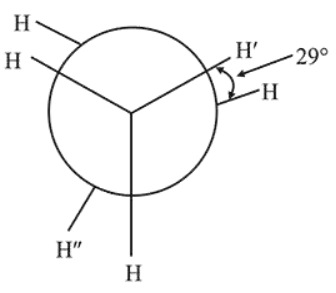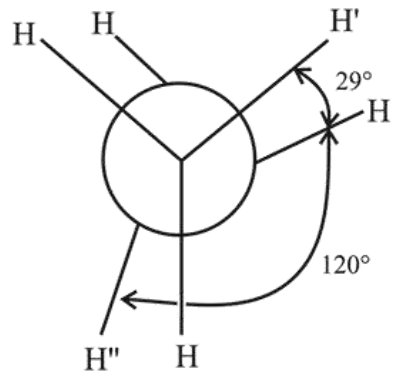# In the following skew conformation of ethane,Question:

In the following skew conformation of ethane, $\mathrm{H}^{\prime}-\mathrm{C}-\mathrm{C}-\mathrm{H}^{\prime \prime}$ dihedral angle is :1. $58^{\circ}$

2. $149^{\circ}$

3. $151^{\circ}$

4. $120^{\circ}$

Correct Option: , 2

Solution:$\therefore$ Angle between $\mathrm{H}^{\prime}$ and $\mathrm{H}^{\prime \prime}=120^{\circ}+29^{\circ}=149^{\circ}$